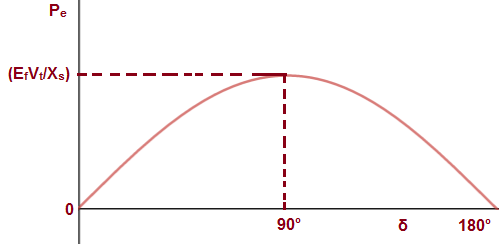# Power Angle Curve of Synchronous Machine

Power Angle Curve of Synchronous Machine is the graphical representation of electrical output with respect to the power angle. As we know, power angle is also known as load angle, therefore it can be said that this curve is graphical representation of electrical output of generator with respect to load angle. In this article, we will discuss power angle curve and its importance.

First of all, we should know the mathematical relation between the electrical output of synchronous machine in terms of load angle to get the graph of power versus load angle.  The electrical output of synchronous generator is given as below.

Pe = (EfVt/Xs)Sinδ

Where Ef, Vt, Xs and δ are no load excitation voltage, generator terminal voltage, generator synchronous reactance and load angle respectively. You are requested to read “Power Flow Equation through an Inductive Load” for getting the detail of derivation part of the above expression of electrical output.

Let us now draw a graph between Pe and load angle δ assuming rest of the parameters to be constant.Above graphical representation of power w.r.t δ is called Power Angle Curve. It can be easily seen from the above graph that it is sinusoidal. Thus power angle curve is sinusoidal.

### Importance of Power Angle Curve

Power Angle Curve tells us about the electrical power output of synchronous machine when power angle δ is varied. It can be seen from this curve that as we increase δ from 0 to 90°, the output increases sinusoidally. But a further increase in power angle δ beyond 90°, the generator electrical output decreases. What does this mean?

This simply means that, the generator electrical output is less than the mechanical input. Therefore, the poles of the machine will start to slip and eventually it will lose synchronism. Thus the machine i.e. generator becomes unstable. Steady state stability limit is the maximum power flows possible through a specific point without lose of synchronism, when the power is increased gradually. Therefore, steady state stability limit of synchronous machine corresponds to power for load angle δ = 90°. To be accurate, it will be (EfVt/Xs).

Not only steady state stability limit rather transient stability limit is also affected by the load angle at which machine is operating. Transient state stability limit is basically the maximum amount of power flow possible without loss of synchronism when a sudden disturbance occurs. The transient stability limit is determined by Equal Area Criteria which uses power angle curve. Thus power angle curve is very important for study of stability limit of synchronous machine.

This site uses Akismet to reduce spam. Learn how your comment data is processed.